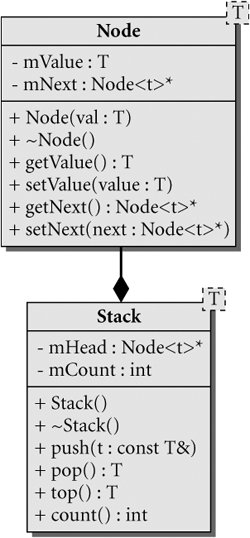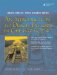# Generics and Templates

C++ supports four distinct categories of types:

1. Primitives: int, char, float, double, etc.
2. Pointers
3. Instances of class/struct
4. Arrays

Because there is no common base type for these four distinct type categories, writing generic functions and classes that can operate on multiple type categories would be very difficult without the use of templates. Templates provide a means for C++ to generate different versions of classes and functions with parameterized types and common behavior. They are distinguished by the use of the keyword template, and a template parameter enclosed in angle brackets <>.

A template parameter differs from a function parameter in that it can be used to pass not only variables and values, but also type expressions.

```template T > class String { ... };
template T, int max > Buffer { ...
T v[max];
};
String  s1;
Buffer  intBuf10;
```

10.1.1. Function Templates

Function templates are used to create type-checked functions which all work on the same pattern. Example 10.1 defines a template function that raises a value of type T to the power exp by repeatedly applying the operator*=.

Example 10.1. src/templates/template-demo.cpp

 ```[ . . . . ] template T power (T a, int exp) { T ans = a; while (--exp > 0) { ans *= a; } return (ans); } ```

When the function is called, as shown in Example 10.2, different function bodies will be automatically generated by the compiler based on the argument types supplied in the function call. Even though the word class is in the template parameter, we can supply a class or a primitive type for T. The only limitation on the type T is that it must be a type for which the operator*= is defined.

Example 10.2. src/templates/template-demo.cpp

 ```[ . . . . ] int main() { Complex z(3,4), z1; Fraction f(5,6), f1; int n(19); z1 = power(z,3); <-- 1 f1 = power(f,4); <-- 2 z1 = power(n, 4); <-- 3 z1 = power(n,5); <-- 4 } ```   (1)First instantiation T is Complex. (2)Second instantiation T is Fraction. (3)Supply an explicit template parameter if the actual argument is not "specific" enough. This results in a call to a function that was already instantiated. (4)Which version gets called?

Each time the compiler sees a template function used for the first time with a specific combination of parameter types, we say the template is instantiated. Subsequent uses of of power(Complex, int) or power(Fraction, int) will be translated into ordinary function calls.

Exercises: Function Templates

 1. Complete Example 10.2. In particular, write a generic Complex and Fraction class, and fix main() so that it works and uses those classes. 2. Write a template version of swap(), based on Example 5.13. Write client code to test it thoroughly. 3. Are there any types for which swap() does not work? 4. Specify the restrictions on the class parameter in your template swap function.

10.1.2. Class Templates

Like functions, classes can also use parameterized types. Class templates are used to generate generic containers of data. The parameter is the answer to the question, "Container of what?" All Qt container classes and, of course, all classes in the Standard Template Library (STL) are parameterized.

We will discuss a homemade example of a template stack class. Figure 10.1 shows a UML diagram of two template classes.

Figure 10.1. Template-based stackUML locates the template parameter in a small offset box in the upper-right corner of the class box. Example 10.3 contains definitions for these classes.

Example 10.3. src/collections/stack/stack.h

 ```[ . . . . ] #include template class Node { public: Node(T invalue): m_Value(invalue), m_Next(0) {} ~Node() ; T getValue() const {return m_Value;} void setValue(T value) {m_Value = value;} Node* getNext() const {return m_Next;} void setNext(Node* next) {m_Next = next;} private: T m_Value; Node* m_Next; }; template Node::~Node() { using namespace qstd; cout << m_Value << " deleted " << endl; if(m_Next) { delete m_Next; } } template class Stack { public: Stack(): m_Head(0), m_Count(0) {} ~Stack() {delete m_Head;} void push(const T& t); T pop(); T top() const; int count() const; private: Node *m_Head; int m_Count; }; ```

Notice that template definitions, shown in Example 10.4 (classes and functions), appear in the header file. This is necessary for the compiler to generate code from a template declaration. Also notice the required template declaration code, template. This code must precede each class or function definition that has a template parameter in its name.

Example 10.4. src/collections/stack/stack.h

 ```[ . . . . ] template void Stack::push(const T& value) { Node *newNode = new Node(value); newNode->setNext(m_Head); m_Head = newNode; ++m_Count; } template T Stack::pop() { Node *popped = m_Head; if (m_Head != 0) { m_Head = m_Head->getNext(); T retval = popped->getValue(); popped->setNext(0); delete popped; --m_Count; return retval; } return 0; } ```

The creation of objects is handled generically in the template function, push(). The destructor for the Node class recursively deletes Node pointers until it reaches one with a zero m_Next pointer. Controlling creation and destruction of Node objects this way enables Stack to completely manage its dynamic memory. Example 10.5 contains some client code to demonstrate these classes.

 This is a consequence of the fact that calling delete on a pointer automatically invokes the destructor associated with that pointer.

Example 10.5. src/collections/stack/main.cpp

 ```#include #include #include "stack.h" int main() { Stack intstack1, intstack2; int val; for(val = 0; val < 4; ++val) { intstack1.push(val); intstack2.push(2 * val); } while (intstack1.count()) { val = intstack1.pop(); qDebug() << val; } Stack stringstack; stringstack.push("First on"); stringstack.push("second on"); stringstack.push("first off"); QString val2; while (stringstack.count()) { val2 = stringstack.pop(); qDebug() << val2; } qDebug() << "Now intstack2 will self destruct."; return 0; } Output: 3 deleted 3 2 deleted 2 1 deleted 1 0 deleted 0 first off deleted "first off" second on deleted "second on" First on deleted "First on" Now intstack2 will self destruct. 6 deleted 4 deleted 2 deleted 0 deleted ```

Exercises: Class Templates

 1 Place the function definitions for Stack in a separate file (stack.cpp), modify the project file appropriately, and then build the app. Explain the results. 2 How general is this application (i.e., what conditions must the class T satisfy in order to be used here)? 3 What limits the size of a Stack? 4 Write a template Queue class and client code to test it.An Introduction to Design Patterns in C++ with Qt 4
ISBN: 0131879057
EAN: 2147483647
Year: 2004
Pages: 268